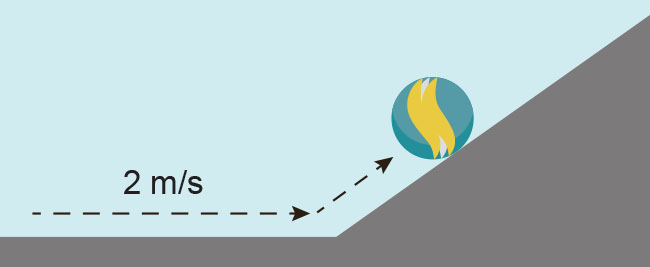Physics

# Problems

PhysicsProblems

### Problems

#### 9.1Work, Power, and the Work–Energy Theorem

14.
A baseball player exerts a force of 100\,\text{N} on a ball for a distance of 0.5\,\text{m} as he throws it. If the ball has a mass of 0.15\,\text{kg}, what is its velocity as it leaves his hand?
1. -36.5\,\text{m/s}
2. -25.8\,\text{m/s}
3. 25.8\,\text{m/s}
4. 36.5\,\text{m/s}
15.

A boy pushes his little sister on a sled. The sled accelerates from 0 to 3.2 m/s . If the combined mass of his sister and the sled is 40.0 kg and 18 W of power were generated, how long did the boy push the sled?

1. 205 s
2. 128 s
3. 23 s
4. 11 s

#### 9.2Mechanical Energy and Conservation of Energy

16.
What is the kinetic energy of a 0.01\,\text{kg} bullet traveling at a velocity of 700\,\text{m/s}?
1. 3.5\,\text{J}
2. 7\,\text{J}
3. 2.45 \times 10^3\,\text{J}
4. 2.45 \times 10^5\,\text{J}
17.A marble is rolling across a flat surface with a velocity of 2 m/s. It begins to roll up a ramp. Ignoring rotational kinetic energy and friction, what will be the vertical height of the marble when it comes to a stop before rolling back down?
1. 0.1\,\text{m}
2. 0.2\,\text{m}
3. 0.4\,\text{m}
4. 2\,\text{m}
18.

The potential energy stored in a compressed spring is $U= 1 2 k x 2 U= 1 2 k x 2$, where k is the force constant and x is the distance the spring is compressed from the equilibrium position. Four experimental setups described below can be used to determine the force constant of a spring. Which one(s) require measurement of the fewest number of variables to determine k? Assume the acceleration due to gravity is known.

1. An object is propelled vertically by a compressed spring.
2. An object is propelled horizontally on a frictionless surface by a compressed spring.
3. An object is statically suspended from a spring.
4. An object suspended from a spring is set into oscillatory motion.
1. I only
2. III only
3. I and II only
4. III and IV only

#### 9.3Simple Machines

19.

A man is using a wedge to split a block of wood by hitting the wedge with a hammer. This drives the wedge into the wood creating a crack in the wood. When he hits the wedge with a force of 400 N it travels 4 cm into the wood. This caused the wedge to exert a force of 1,400 N sideways increasing the width of the crack by 1 cm . What is the efficiency of the wedge?

1. 0.875 percent
2. 0.14
3. 0.751
4. 87.5 percent
20.

An electrician grips the handles of a wire cutter, like the one shown, 10 cm from the pivot and places a wire between the jaws 2 cm from the pivot. If the cutter blades are 2 cm wide and 0.3 cm thick, what is the overall IMA of this complex machine?1. 1.34
2. 1.53
3. 33.3
4. 33.5
Order a print copy

As an Amazon Associate we earn from qualifying purchases.